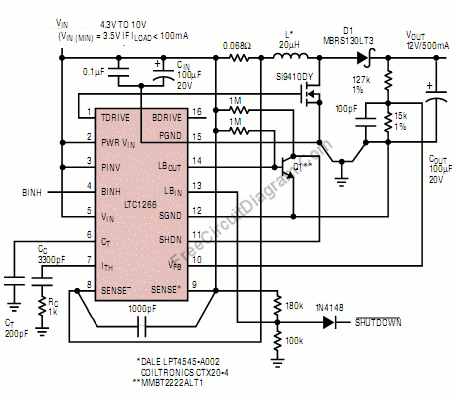## TPS108x Versatile Boost Converter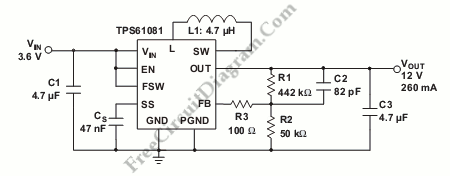This is Versatile Boost Converter circuit using TPS108x. The TPS1081 has chosen because it is suitable for application that requires up to 20 V and 100 mA to drive each column of a passive-matrix OLED (PMOLED). the TPS61080 is suitable for application that requires less than 10 V and only tens of milliamps per column for the active-matrix OLED (AMOLED). […]

## Versatile Boost Converter with TPS6108x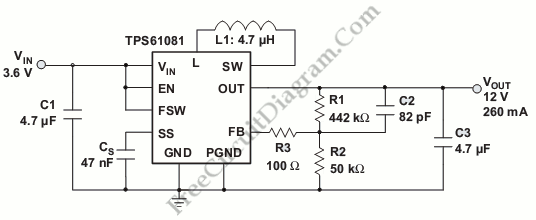There are two types of highly integrated boost converters, the TPS61081 and  TPS61080.  Both of them require low input voltage of 2.5V and the output is adjustable up to 27 V. The TPS61080’s current limit rating of the integrated power switches is 0.5 A and 1.3 A for TPS61081. Here is the circuit : It is useful for industrial application […]

## Miniature Voltage Isolation Provides Positive and Negative Supply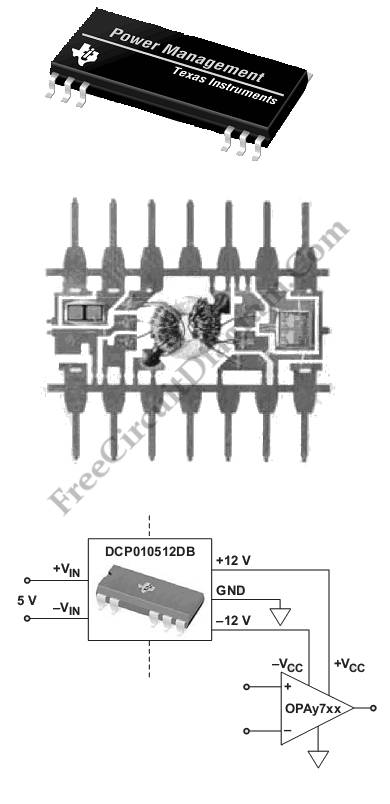The development of miniature low-power (<2-W) DC/DC converters is growing rapidly because of the hot growth in distributed-power architecture. This application minimize the converter’s impact onboard space. This device is very useful for safety-critical applications such as medical equipment, and telecommunications, and industrial applications because it provides point-of-use isolated power conversion for analog circuitry. In addition, this device can be […]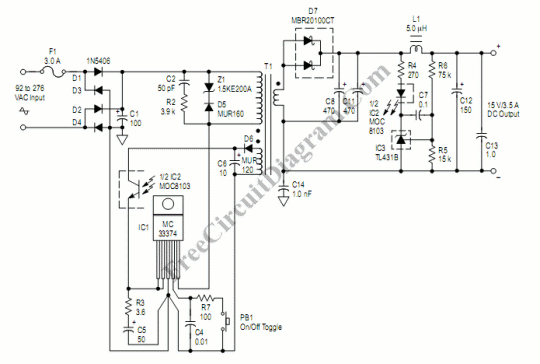This SMPS AC-DC converter gives good efficiency. Test data shows efficiency of 83.2% at 115 Vac input and 6A output current, and 85.4% at 230 Vac input and 6A output current. Moreover, the input voltage range is universal, accommodating 92 to 276 Vac variation with only 24 mV change in the output voltage. Here is the schematic diagram of the […]

## 450W Power Factor Controller with Universal Input Voltage Range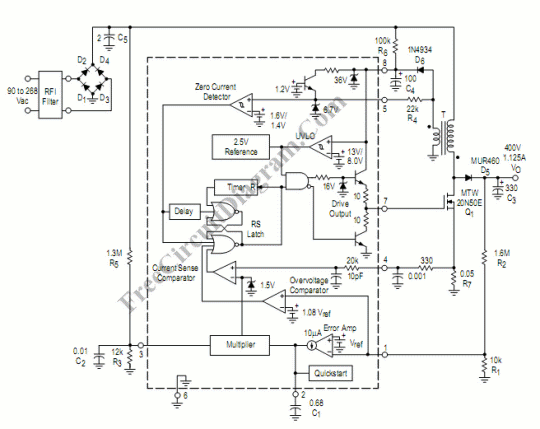This power factor controller accept voltage from 90 to 268 Vac,  that’s why we call it power factor controller with universal input, since any standards of mains supply  in almost any country would fall within that range. This power factor controller is capable of handling up to 450W load. Here is the schematic diagram of the power factor controller circuit: […]

## Constant Current Constant Voltage SMPS (Switch Mode Power Supply)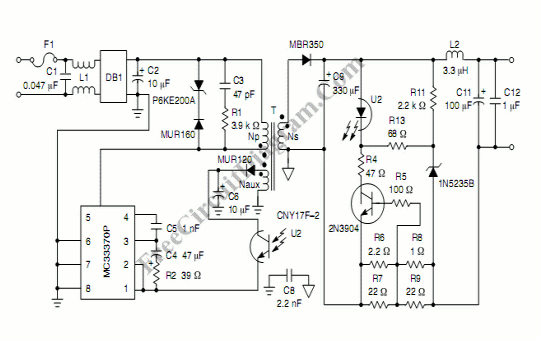This constant current constant voltage  SMPS can be used to efficiently charge a battery. This circuit gives 7.2V at constant voltage mode, and 600 mA at constant current mode. Here is the schematic diagram of the circuit: The mechanism is simple, when the load impedance is high, then the output voltage will be constant at 7.2V.  When the load impedance […]